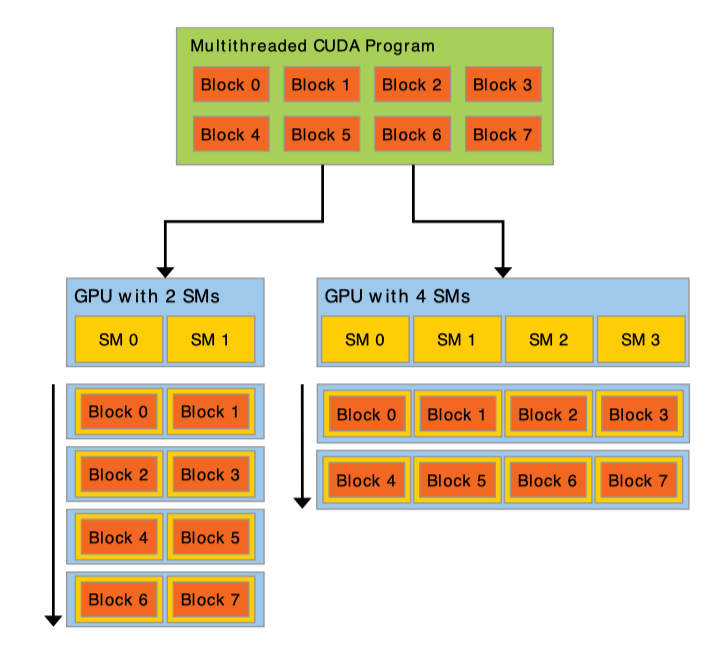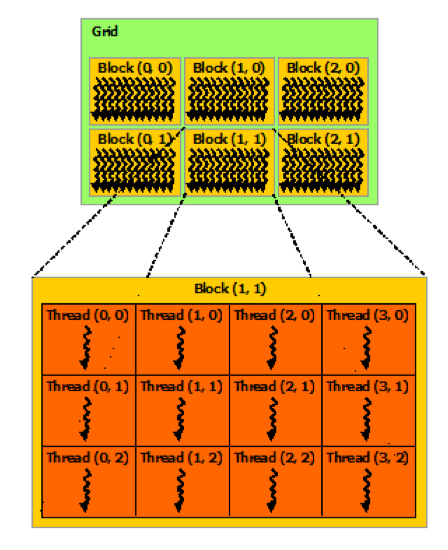# CUDA C++ 编程指引：编程模型 | CUDA# 内核函数

__global__ void VecAdd(float* A, float* B, float* C) {
int i = threadIdx.x; C[i] = A[i] + B[i];
}

int main() {
...
...
}

# 线程

__global__ void MatAdd(float A[N][N], float B[N][N], float C[N][N]) {
C[i][j] = A[i][j] + B[i][j];
}

int main() {
...
int numBlocks = 1;
...
}

__global__ void MatAdd(float A[N][N], float B[N][N], float C[N][N]) {
int i = blockIdx.x * blockDim.x + threadIdx.x;
int j = blockIdx.y * blockDim.y + threadIdx.y;
if (i < N && j < N)
C[i][j] = A[i][j] + B[i][j];
}

int main() {
...
}